# Android：修图技术之瘦脸效果的实现(drawBitmapMesh)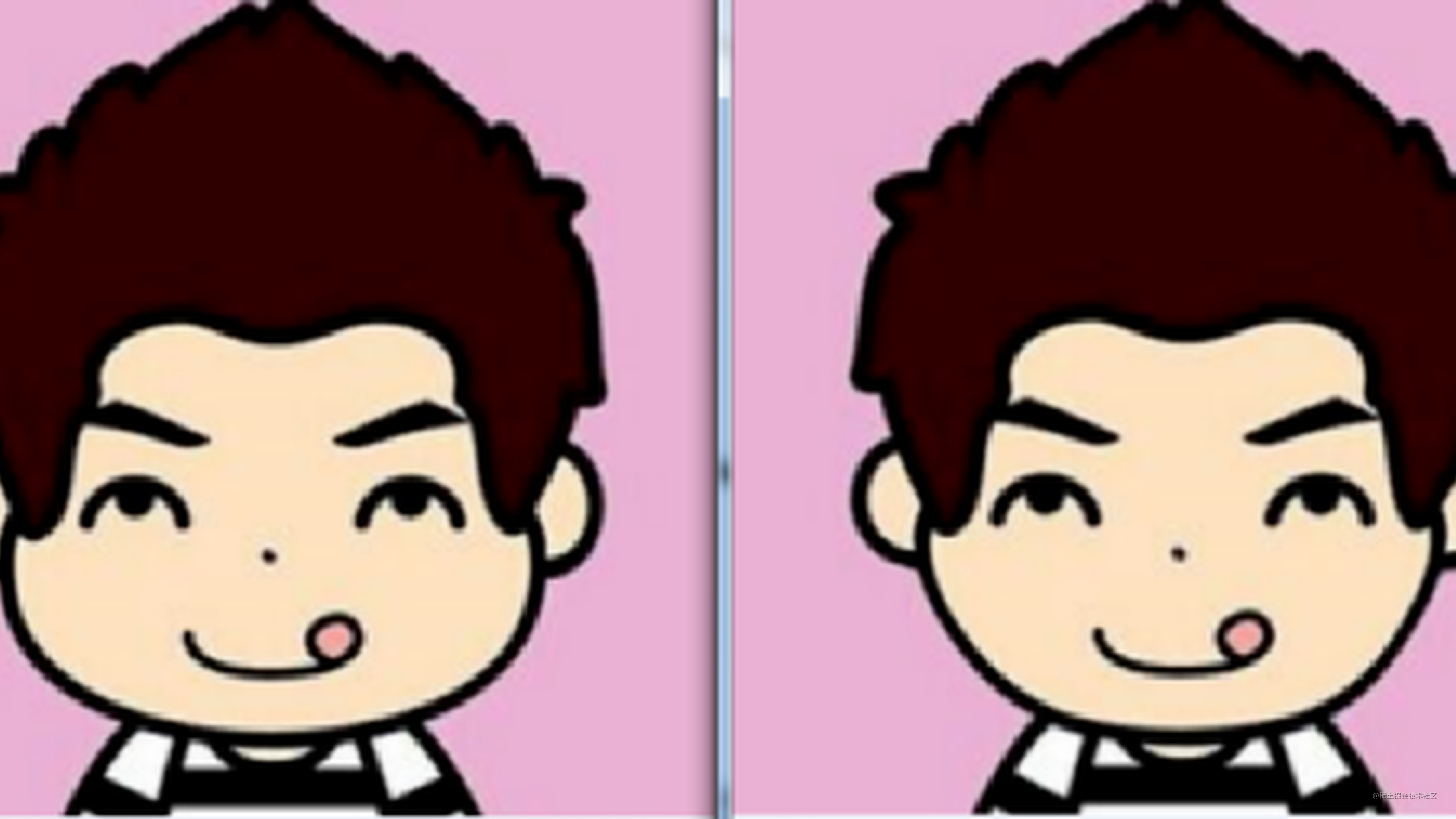### 一、初识Canvas.drawBitmapMesh()

##### 1、方法介绍分析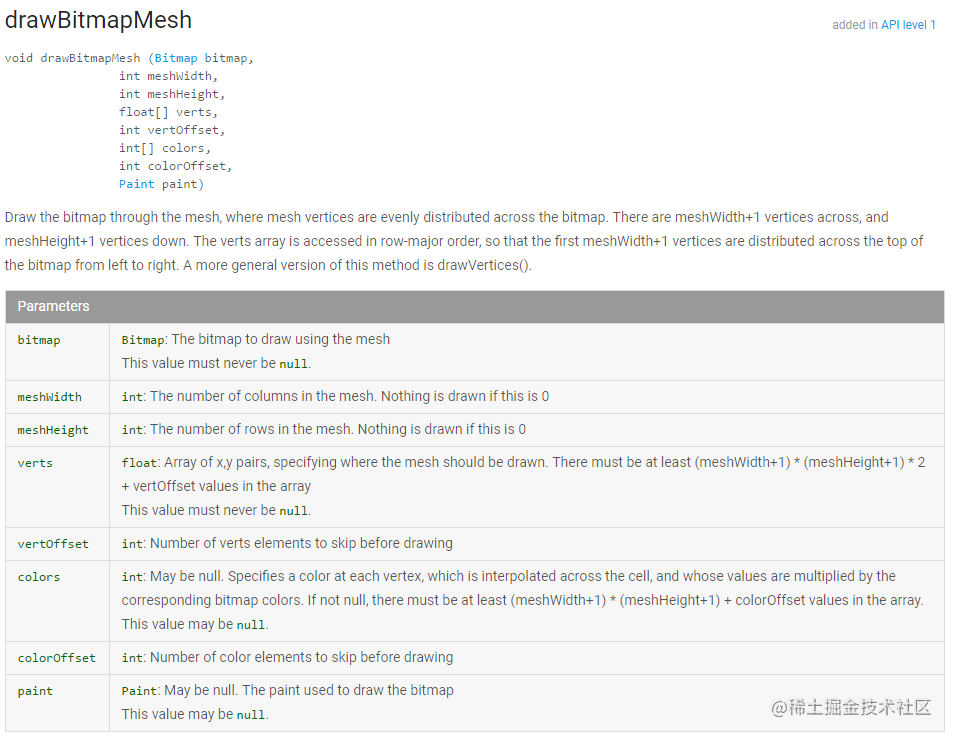drawBitmapMesh方法

• bitmap：将要扭曲的图像
• meshWidth：控制在横向上把该图像划成多少格
• meshHeight：控制在纵向上把该图像划成多少格
• verts：网格交叉点坐标数组，长度为(meshWidth + 1) * (meshHeight + 1) * 2
• vertOffset：控制verts数组中从第几个数组元素开始才对bitmap进行扭曲

Android 中的 drawBitmapMesh() 方法与操纵像素点来改变色彩的原理类似。只不过是把图像分成一个个的小块，然后通过改变每一个图像块来改变整个图像。来看看下面这张经典的图像对比：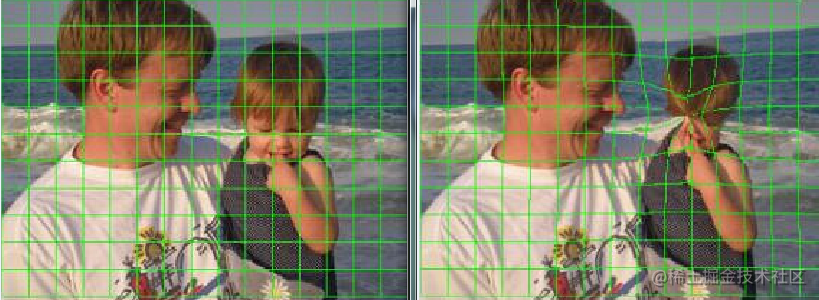drawBitmapMesh效果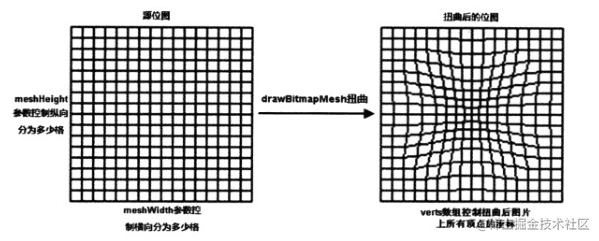Canvas.drawBitmapMesh()
##### 2、方法代码实现

``````    //将图像分成多少格
private int WIDTH = 200;
private int HEIGHT = 200;
//交点坐标的个数
private int COUNT = (WIDTH + 1) * (HEIGHT + 1);
//用于保存COUNT的坐标
//x0, y0, x1, y1......
private float[] verts = new float[COUNT * 2];
//用于保存原始的坐标
private float[] orig = new float[COUNT * 2];

private void initView() {
int index = 0;
Bitmap mBitmap = BitmapFactory.decodeResource(getResources(), R.drawable.test00);
float bmWidth = mBitmap.getWidth();
float bmHeight = mBitmap.getHeight();

for (int i = 0; i < HEIGHT + 1; i++) {
float fy = bmHeight * i / HEIGHT;
for (int j = 0; j < WIDTH + 1; j++) {
float fx = bmWidth * j / WIDTH;
//X轴坐标 放在偶数位
verts[index * 2] = fx;
orig[index * 2] = verts[index * 2];
//Y轴坐标 放在奇数位
verts[index * 2 + 1] = fy;
orig[index * 2 + 1] = verts[index * 2 + 1];
index += 1;
}
}
}复制代码``````

``````    @Override
protected void onDraw(Canvas canvas) {
super.onDraw(canvas);
canvas.drawBitmapMesh(mBitmap, WIDTH, HEIGHT, verts, 0, null, 0, null);
}复制代码``````

### 二、实现瘦脸效果

##### １、算法提及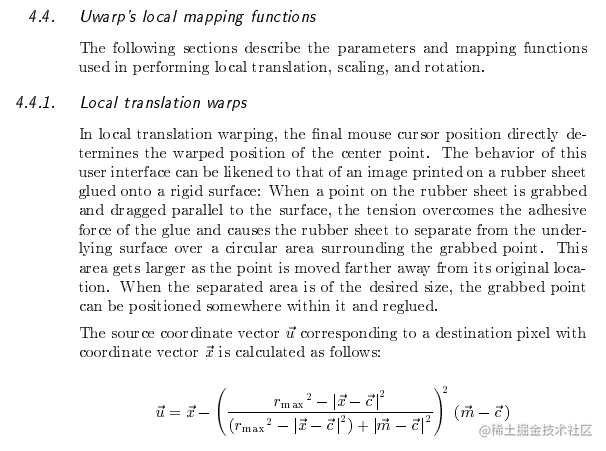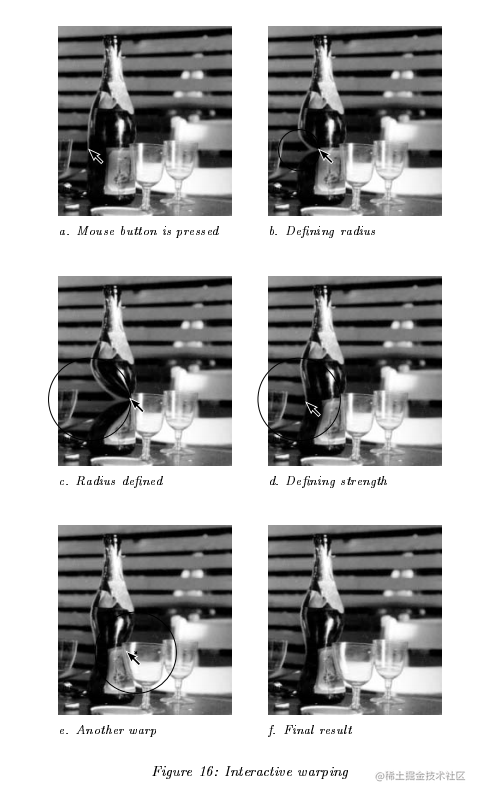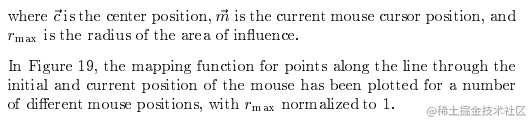##### 2、算法分析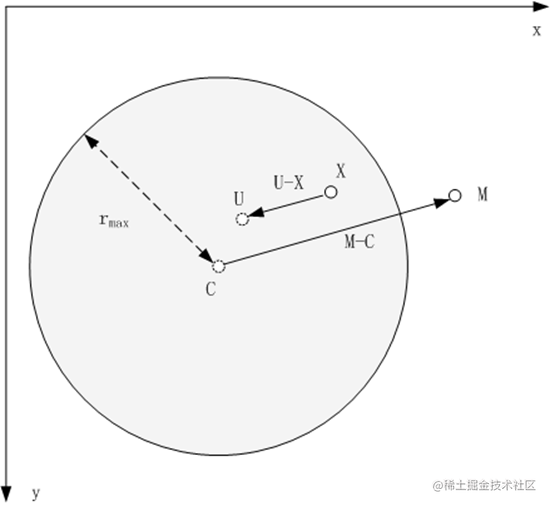• 只有圆形选区内的图像才进行变形（这里需要自己用代码控制一下）
• 拖动距离 MC 越大变形效果越明显（这里需要自己用代码控制一下，下面我会给大家讲讲）
• 越靠近圆心，变形越大，越靠近边缘的变形越小，边界处无变形（算法公式已经实现）
• 变形是平滑的（算法公式已经实现）

A(X1,Y1) ； B(X2,Y2)，则：
A+B=（X1+X2，Y1+Y2），A-B=（X1-X2，Y1-Y2）

##### 3、算法的代码实现

``````    @Override
public boolean onTouchEvent(MotionEvent event) {
switch (event.getAction()) {
case MotionEvent.ACTION_DOWN:
startX = event.getX();
startY = event.getY();
break;
case MotionEvent.ACTION_UP:
//调用warp方法根据触摸屏事件的坐标点来扭曲verts数组
warp(startX, startY, event.getX(), event.getY());
break;
}
return true;
}复制代码``````

``````//作用范围半径
private int r = 100;复制代码``````

``````    private void warp(float startX, float startY, float endX, float endY) {

//计算拖动距离
float ddPull = (endX - startX) * (endX - startX) + (endY - startY) * (endY - startY);
float dPull = (float) Math.sqrt(ddPull);
//文献中提到的算法，并不能很好的实现拖动距离 MC 越大变形效果越明显的功能，下面这行代码则是我对该算法的优化
dPull = screenWidth - dPull >= 0.0001f ? screenWidth - dPull : 0.0001f;

for (int i = 0; i < COUNT * 2; i += 2) {
//计算每个坐标点与触摸点之间的距离
float dx = verts[i] - startX;
float dy = verts[i + 1] - startY;
float dd = dx * dx + dy * dy;
float d = (float) Math.sqrt(dd);

//文献中提到的算法同样不能实现只有圆形选区内的图像才进行变形的功能，这里需要做一个距离的判断
if (d < r) {
//变形系数，扭曲度
double e = (r * r - dd) * (r * r - dd) / ((r * r - dd + dPull * dPull) * (r * r - dd + dPull * dPull));
double pullX = e * (endX - startX);
double pullY = e * (endY - startY);
verts[i] = (float) (verts[i] + pullX);
verts[i + 1] = (float) (verts[i + 1] + pullY);
}
}
invalidate();
}复制代码``````Demo效果

##### 4、补充

###### 4.1.添加作用范围圆形的显示和瘦脸拖动方向的显示

``````    //是否显示变形圆圈
private boolean showCircle;
//是否显示变形方向
private boolean showDirection;

@Override
protected void onDraw(Canvas canvas) {
super.onDraw(canvas);
canvas.drawBitmapMesh(mBitmap, WIDTH, HEIGHT, verts, 0, null, 0, null);
if (showCircle) {
canvas.drawCircle(startX, startY, r, circlePaint);
}
if (showDirection) {
canvas.drawLine(startX, startY, moveX, moveY, directionPaint);
}
}复制代码``````

``````    @Override
public boolean onTouchEvent(MotionEvent event) {
switch (event.getAction()) {
case MotionEvent.ACTION_DOWN:
//绘制变形区域
startX = event.getX();
startY = event.getY();
showCircle = true;
invalidate();
break;
case MotionEvent.ACTION_MOVE:
//绘制变形方向
moveX = event.getX();
moveY = event.getY();
showDirection = true;
invalidate();
break;
case MotionEvent.ACTION_UP:
showCircle = false;
showDirection = false;

//调用warp方法根据触摸屏事件的坐标点来扭曲verts数组
warp(startX, startY, event.getX(), event.getY());
break;
}
return true;
}复制代码``````
###### 4.2.添加一键复原的按钮

``````    /**
* 一键恢复
*/
public void resetView() {
for (int i = 0; i < verts.length; i++) {
verts[i] = orig[i];
}
onStepChangeListener.onStepChange(true);
invalidate();
}

public void setOnStepChangeListener(IOnStepChangeListener onStepChangeListener) {
this.onStepChangeListener = onStepChangeListener;
}

public interface IOnStepChangeListener {
void onStepChange(boolean isEmpty);
}复制代码``````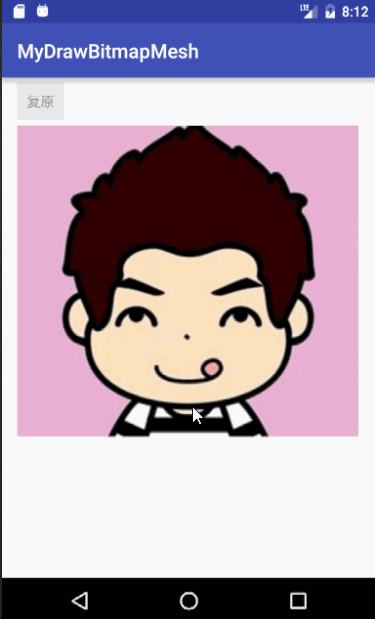MyDrawBitmapMeshDemo

### 后续

1. Demo中还有很多可以完善的细节，这里只做原理分析，感兴趣的同学可以继续完善，比如，变形区域的动态设置，记录每一次变形的数组值用于撤销上一步操作，等等。同样的，这里不仅仅可以瘦脸，还可以瘦各种地方。如果需要做拉伸处理，只需要将 verts[] 数组里的元素做相应的处理即可。

2. 如果对图像滤镜效果感兴趣，可以看看我的上一篇文章 Android：修图技术之滤镜效果实现及原理分析——ColorMatrix

3. Demo 下载地址

Android

Android